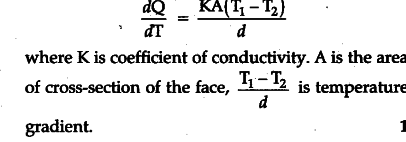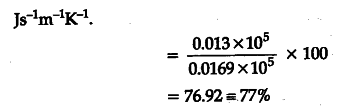# What is conduction ? Give an expression for thermal conductivity. Define coefficient of thermal conductivity

Conduction is the process of flow of heat from one point to another through a substance without the actual transfer of particle.
Rate of flow of heat,Coefficient of thermal conductivity (K) is defined as the amount of heat flowing in a second between two opposite faces of a cube of side 1 m kept at a temperature difference of 1 K. S.I. unit of K is